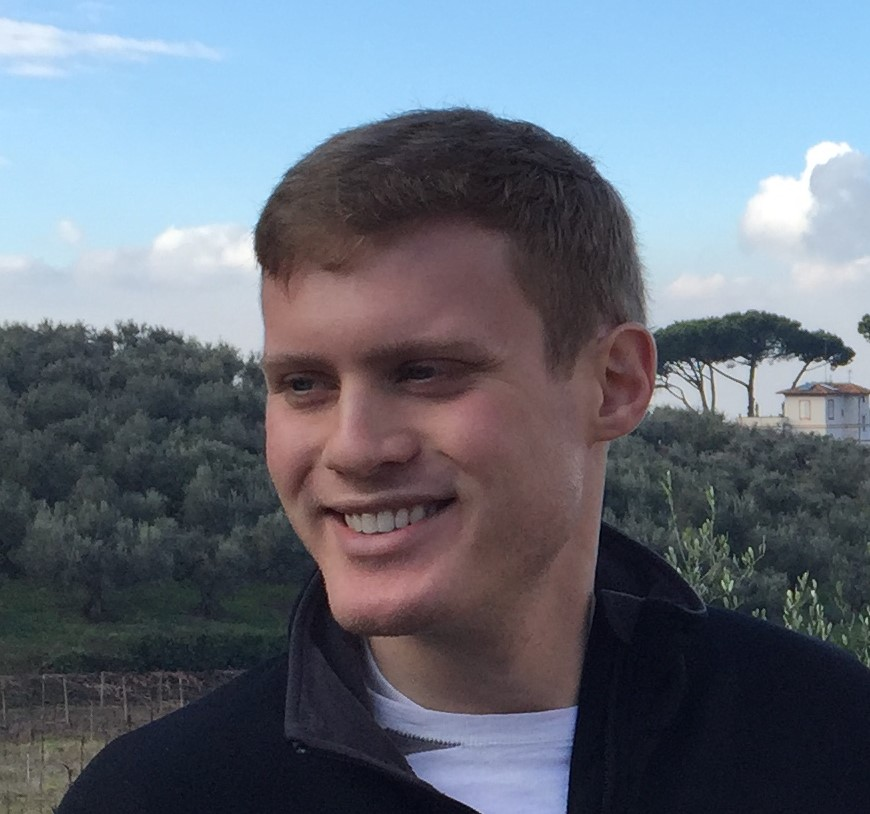I am a computer science Ph.D. student at the University of Wisconsin-Madison. My advisor is Thomas Reps.

## Papers

##### Exact and Approximate Methods for Proving Unrealizability of Syntax-Guided Synthesis Problems (arxiv version)To appear @PLDI 2020

Qinheping Hu, John Cyphert, Loris D' Antoni, and Thomas Reps

We again consider the problem of automatically establishing that a given syntax-guided-synthesis (SyGuS) problem is unrealizable. However, in this work we present an algorithm for exactly solving a set of equations that result from SyGuS problems over linear integer arithmetic (LIA) and LIA with conditionals (CLIA), thereby showing that LIA and CLIA SyGuS problems over finitely many examples are decidable. We implemented the proposed technique and algorithms in a tool called Nay. Nay can prove unrealizability for 70/132 existing SyGuS benchmarks, with running times comparable to those of the state-of-the-art tool Nope. Moreover, Nay can solve 11 benchmarks that Nope cannot solve.

##### Templates and Recurrences: Better Together (arxiv version)To appear @PLDI 2020

Jason Breck, John Cyphert, Zachary Kincaid, and Thomas Reps

This paper is the confluence of two streams of ideas in the literature on generating numerical invariants, namely: (1) template-based methods, and (2) recurrence-based methods. In this paper, we combine these two approaches and obtain a technique that uses templates in which the unknowns are functions rather than numbers, and the constraints on the unknowns are recurrences. The technique synthesizes invariants involving polynomials, exponentials, and logarithms, even in the presence of arbitrary control-flow, including any combination of loops, branches, and (possibly non-linear) recursion. For instance, it is able to show that (i) the time taken by merge-sort is $$O(n\log(n))$$, and (ii) the time taken by Strassen's algorithm is $$O(n^{log_2(7)})$$.

##### Proving Unrealizability for Syntax-Guided Synthesis@CAV 2019 [ slides ]

Qinheping Hu, Jason Breck, John Cyphert, Loris D' Antoni, and Thomas Reps

We consider the problem of automatically establishing that a given syntax-guided-synthesis ($$SyGuS$$) problem is unrealizable (i.e., has no solution). We reduce the unrealizability problem to a reachability problem by encoding the synthesis problem's grammar $$G$$ as a nondeterministic program $$P_G$$, such that, if a standard program-analysis tool can establish that a certain assertion in $$P_G$$ always holds, then the synthesis problem is unrealizable.

##### Refinement of Path Expressions for Static Analysis@POPL 2019 [ slides ]

John Cyphert, Jason Breck, Zachary Kincaid, and Thomas Reps

Algebraic program analyses compute information about a program's behavior by first (a) computing a valid path expression and then (b) interpreting the path expression in a semantic algebra that defines the analysis. There are an infinite number of different regular expressions that qualify as valid path expressions, which raises the question: ''Which one should we choose?'' While any choice yields a sound result, for many analyses the choice can have a drastic effect on the precision of the results obtained. In this paper, we develop an algorithm that takes as input a valid path expression $$E$$, and returns a valid path expression $$E'$$ that is guaranteed to yield analysis results that are at least as good as those obtained using $$E$$.

##### Closed Forms for Numerical Loops@POPL 2019 [ slides ]

Zachary Kincaid, Jason Breck, John Cyphert, and Thomas Reps

This paper investigates the problem of reasoning about non-linear behavior of simple numerical loops. This paper characterizes when linear loops have closed forms in simpler theories that are more amenable to automated reasoning. The algorithms for computing closed forms described in the paper avoid the use of algebraic numbers, and produce closed forms expressed using polynomials and exponentials over rational numbers. We show that the logic for expressing these closed forms is decidable. We also show that the procedure for computing closed forms for this class of numerical loops can be used to over-approximate the behavior of arbitrary numerical programs (with unrestricted control flow, non-deterministic assignments, and recursive procedures).

##### Non-Linear Reasoning for Invariant Synthesis@POPL 2018 [ slides ]

Zachary Kincaid, John Cyphert, Jason Breck, and Thomas Reps

This paper presents a method for generating non-linear invariants for general loops (conditional branches, nested loops, non-deterministic assignments, etc.) by analyzing recurrence relations. The key components are an abstract domain for reasoning about non-linear arithmetic, a semantics-based method for extracting recurrence relations from loop bodies, and a recurrence solver that avoids closed forms that involve complex or irrational numbers.

## Workshops

##### Guarantees in Program Synthesis@SYNT 2019

Qinheping Hu, Jason Breck, John Cyphert, Loris D' Antoni, and Thomas Reps

## Some Things

• I completed a set of classes to get my Ph.D. minor in economics.
• I gave a series of lectures to the madPL seminar on the basics of category theory. You can view some typeset notes I am very proud of here. The last set of notes is incomplete. I will try and finish it sometime in the future.
• I am originally from Shelby, Ohio. I received my undergraduate degree from The Ohio State University in 2016.
• According to spotify, my top artists include Jimi Hendrix, Weezer, Led Zeppelin, Childish Gambino, Eric B. & Rakim, Miles Davis, Johnny Cash, Lady Gaga, The White Stripes, and Daft Punk.
• I like playing the guitar in my free time. I am trying to get better at blues improvisation, so if anyone has good resources let me know.